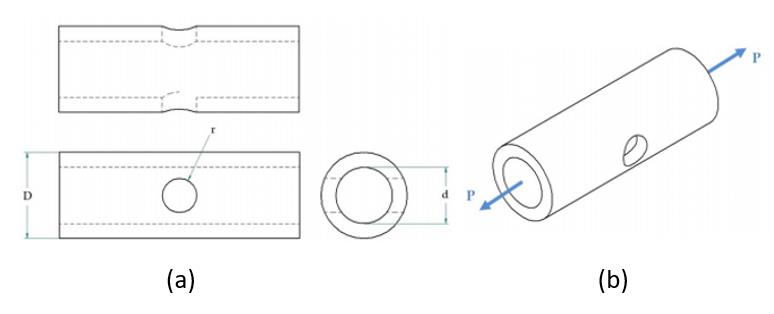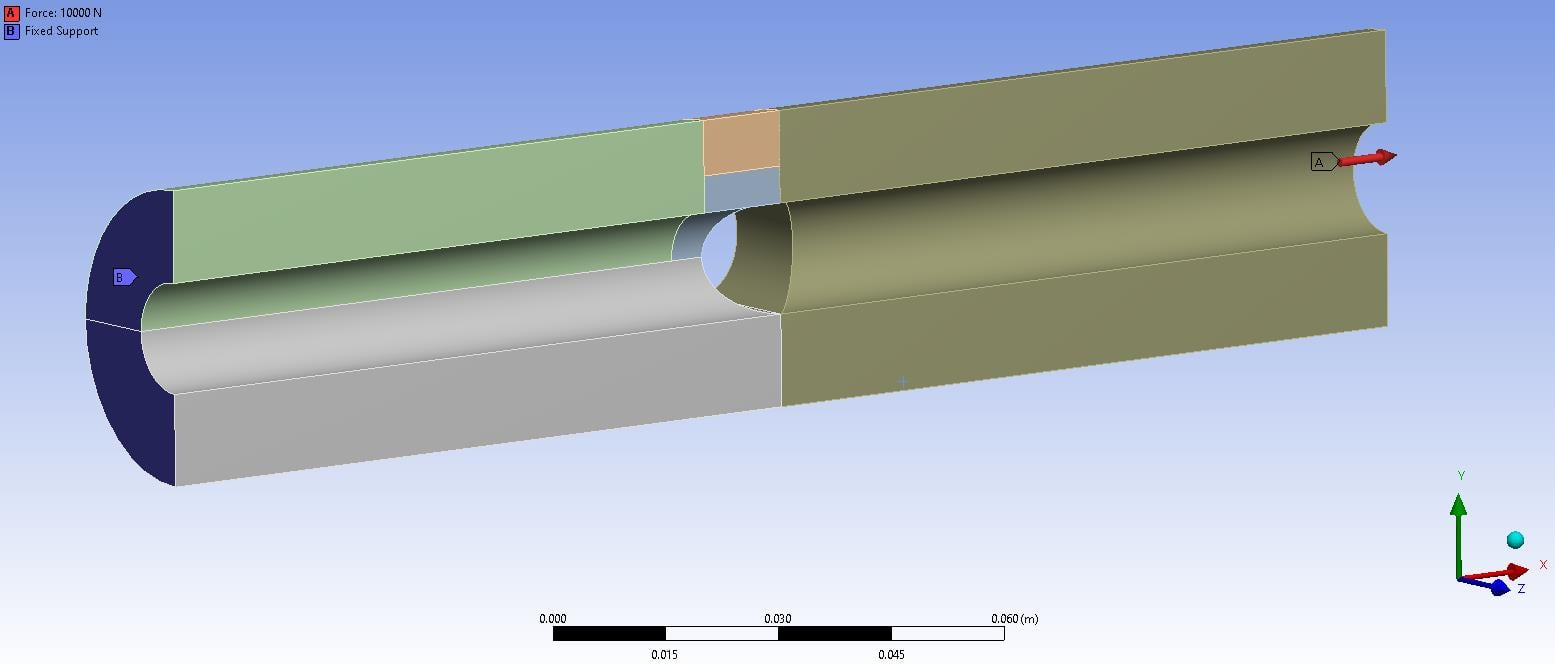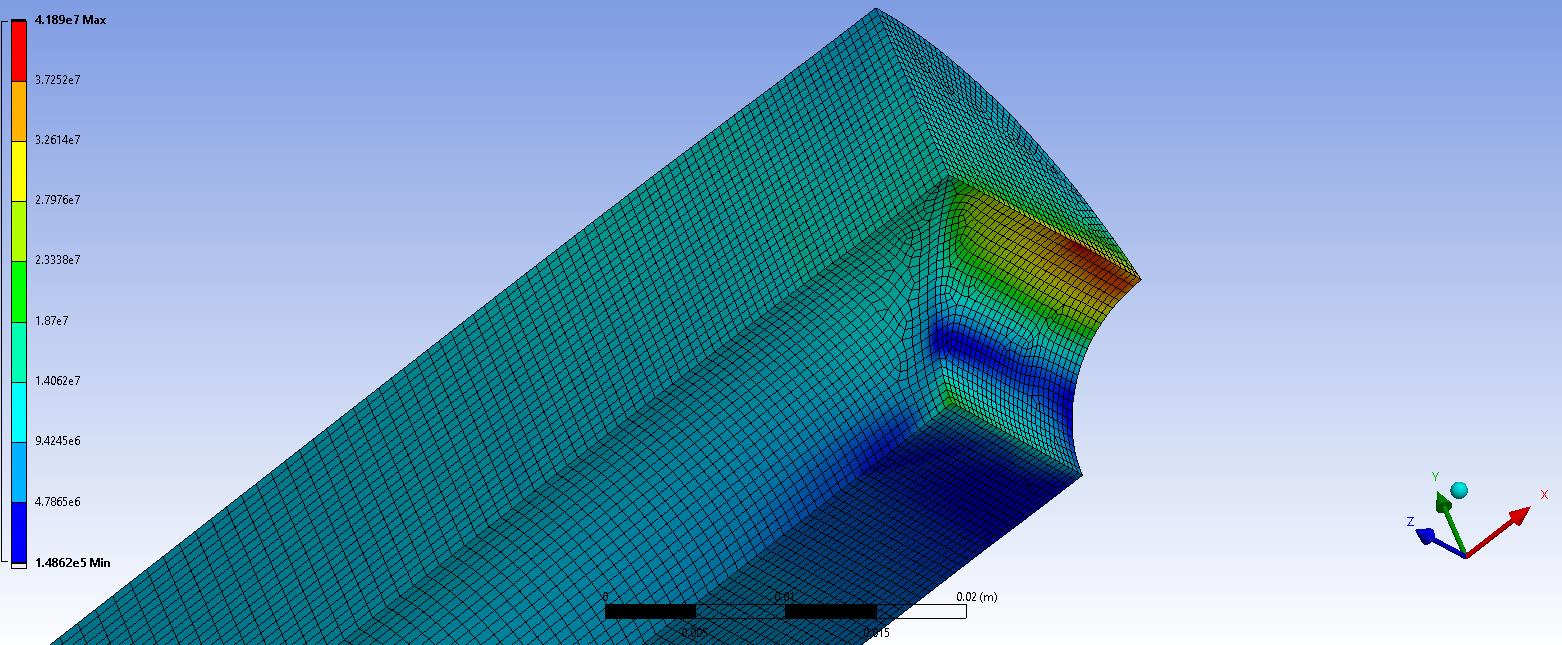## General Mechanical

Topics relate to Mechanical Enterprise, Motion, Additive Print and more

•Jagocullen123
Subscriber

I was given a brief for a steel shaft with a transverse hole under simple tension as shown in the problem descriptor below.The aim of this report is to find the effects of applying a tensional load to a steel shaft with a transverse hole. The material of the shaft had an average yield stress of 400 MPa. A finite element analysis was performed using the parameters provided in the brief, to find what effects applying a load would have on the geometry.

The geometry analysed was as shown in quarter model image as shown below:The  final image shows the vonmises stress which is 41.89 MPa, theoretical value was calculated to be 41.62 MPa:However, this geometry should exceed 400 MPa as detailed in the brief to make changes to bring the maximum vonMises under the maximum yield stress. The tensional force applied is 10 kN.

Any solutions as to why the values seem to be off by a factor of 10?

•peteroznewman
Subscriber

You say that the model had 41.89 MPa and a theoretical value was 41.62 MPa.

How can you also say this geometry should exceed 400 MPa?

If you want that then increase the tension force by a factor of 10.

•jagocullen
Subscriber
In the brief of the assignment a maximum yield was given of 400 MPa, the geometry is to exceed this as the next step is to use parameter optimisation to reduce the vonmises stress below 400 MPa.

The force is 10 kN and is set, so I cannot increase this force by a factor of 10.

As I have model a quarter of the full geometry does the stress have to be multiplied?
•peteroznewman
Subscriber

I'm guessing that you have the same project as this member?

Okay, you can perform a simple parameter sweep on the wall thickness and plot peak stress vs wall thickness and find the value when the stress crosses the 400 MPa line. If you add Parameters in Design Modeler or SpaceClaim, you can automate the parameter sweep in Mechanical.

If you have an equation that can compute the theoretical stress from the geometry, why not plot the stress as the wall thickness changes and not bother to use ANSYS at all?

If the full circle tube has a 10 kN tension load, then a half circle model has 5 kN and a quarter circle model has 2.5 kN.  You can also slice the tube length in half and use symmetry through the center of the length, but that has no effect on the tension force on the end.

•jagocullen
Subscriber
Correct, sorry for the mix up I used a different account by mistake.

Here is the brief of the assignment:

Given the properties for the particular grade of steel from which the plate is manufactured: ? = 7750 Kg/m3, E
= 207 GPa and ? = 0.3, use a finite element analysis (FEA) to determine the location and the magnitude of the
Assuming an average yield stress for steel of 400 MPa, comment on whether the plate is likely to experience
permanent deformation and/or failure as a result of the applied loading. You are required to validate your
finite element results against theoretical results using elementary stress/strain theory and stress concentration
factors. (Use stress in the axial direction along the shaft to validate the results).
Finally, you are required to suggest a geometrical design change (excluding removing the transverse hole)
that may be implemented to reduce the maximum vonMises stress in the shaft. Demonstrate that this design
change does indeed reduce stress by performing an additional FEA that incorporates the suggested design
change. Directly compare the results from the two FEA’s in a table/graph.

The problem I face is the calculations both theoretical and analytical are matching, however in the context of the report values in the brief they would appear to be off by a factor of 10.

I have no problem with the parameters in design modeller, I just feel my calculations are not in line with what is expected?

Does that make better sense?

Apologises for the long post, just hoping to give you a better understanding of the issue I face
•peteroznewman
Subscriber

"Comment on whether the plate is likely to experience permanent deformation and/or failure as a result of the applied loading."

The answer is no, there is no risk of permanent deformation, the Safety Factor is about 10.

As to failure, that depends on whether the load is cyclic and a fatigue failure is possible.

•jagocullen
Subscriber
Thanks for your information and help on the matter.

I will further investigate the cyclic and fatigue failure to see if it produces a result that exceed the maximum value.